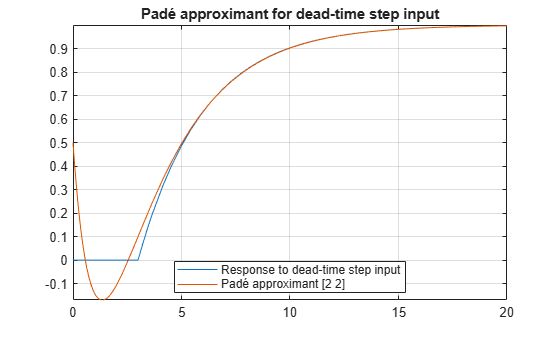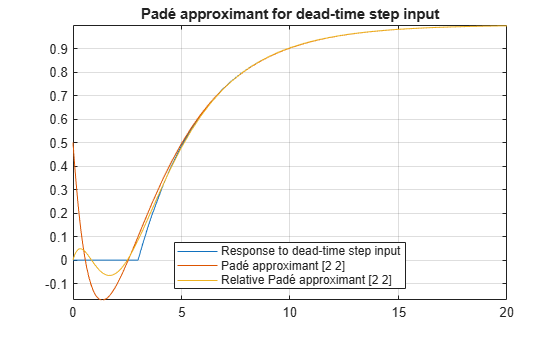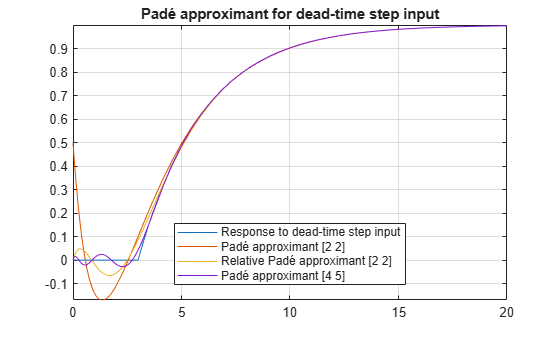## Padé Approximant of Time-Delay Input

This example shows how to use a Padé approximant in control system theory to model time delays in the response of a first-order system. Time delays arise in systems such as chemical and transport processes where there is a delay between the input and the system response. When these inputs are modeled, they are called dead-time inputs.

This example uses Symbolic Math Toolbox™ to solve for the transfer function of a first-order system and find the system response to dead-time step input using Padé approximant. This example performs calculations symbolically to obtain analytic results.

### Introduction

The Padé approximant of order `[m, n]` approximates the function `f(x)` around $x={x}_{0}$ as

`$\frac{{a}_{0}+{a}_{1}\phantom{\rule{0.16666666666666666em}{0ex}}\left(x-{x}_{0}\right)+\cdots +{a}_{m}\phantom{\rule{0.16666666666666666em}{0ex}}\left(x-{x}_{0}{\right)}^{m}}{1+{b}_{1}\phantom{\rule{0.16666666666666666em}{0ex}}\left(x-{x}_{0}\right)+\cdots +{b}_{n}\phantom{\rule{0.16666666666666666em}{0ex}}\left(x-{x}_{0}{\right)}^{n}}.$`

The Padé approximant is a rational function formed by a ratio of two power series. Because it is a rational function, it is more accurate than the Taylor series in approximating functions with poles. The Padé approximant is represented by the Symbolic Math Toolbox™ function `pade`.

When a pole or zero exists at the expansion point $x={x}_{0}$, the accuracy of the Padé approximant decreases. To increase accuracy, use an alternative form of the Padé approximant which is

`$\frac{\left(x-{x}_{0}{\right)}^{p}\left({a}_{0}+{a}_{1}\phantom{\rule{0.16666666666666666em}{0ex}}\left(x-{x}_{0}\right)+\cdots +{a}_{m}\phantom{\rule{0.16666666666666666em}{0ex}}\left(x-{x}_{0}{\right)}^{m}\right)}{1+{b}_{1}\phantom{\rule{0.16666666666666666em}{0ex}}\left(x-{x}_{0}\right)+\cdots +{b}_{n}\phantom{\rule{0.16666666666666666em}{0ex}}\left(x-{x}_{0}{\right)}^{n}}.$`

The `pade` function returns the alternative form of the Padé approximant when you set the `OrderMode` input argument to `Relative`.

### Find Transfer Function of First-Order System

The behavior of a first-order system is described by this differential equation

`$\tau \frac{dy\left(t\right)}{dt}+y\left(t\right)=ax\left(t\right).$`

Enter the differential equation in MATLAB®.

```syms tau a x(t) y(t) xS(s) yS(s) H(s) tmp F = tau*diff(y)+y == a*x;```

Find the Laplace transform of `F` using `laplace`.

`F = laplace(F,t,s)`
`F = $\mathrm{laplace}\left(y\left(t\right),t,s\right)-\tau \left(y\left(0\right)-s \mathrm{laplace}\left(y\left(t\right),t,s\right)\right)=a \mathrm{laplace}\left(x\left(t\right),t,s\right)$`

Assume the response of the system at `t = 0` is `0`. Use `subs` to substitute for `y(0) = 0`.

`F = subs(F,y(0),0)`
`F = $\mathrm{laplace}\left(y\left(t\right),t,s\right)+s \tau \mathrm{laplace}\left(y\left(t\right),t,s\right)=a \mathrm{laplace}\left(x\left(t\right),t,s\right)$`

To collect common terms, use `simplify`.

`F = simplify(F)`
`F = $\left(s \tau +1\right) \mathrm{laplace}\left(y\left(t\right),t,s\right)=a \mathrm{laplace}\left(x\left(t\right),t,s\right)$`

For readability, replace the Laplace transforms of `x(t)` and `y(t)` with `xS(s)` and `yS(s)`.

`F = subs(F,[laplace(x(t),t,s) laplace(y(t),t,s)],[xS(s) yS(s)])`
`F = $\mathrm{yS}\left(s\right) \left(s \tau +1\right)=a \mathrm{xS}\left(s\right)$`

The Laplace transform of the transfer function is `yS(s)/xS(s)`. Divide both sides of the equation by `xS(s)` and use subs to replace `yS(s)/xS(s)` with `H(s)`.

```F = F/xS(s); F = subs(F,yS(s)/xS(s),H(s))```
`F = $H\left(s\right) \left(s \tau +1\right)=a$`

Solve the equation for `H(s)`. Substitute for `H(s)` with a dummy variable, solve for the dummy variable using solve, and assign the solution to `Hsol(s)`.

```F = subs(F,H(s),tmp); Hsol(s) = solve(F,tmp)```
```Hsol(s) =  $\frac{a}{s \tau +1}$```

### Find Response of System to Time-Delayed Step Input

The input to the first-order system is a time-delayed step input. To represent a step input, use `heaviside`. Delay the input by three time units. Find the Laplace transform using `laplace`.

```step = heaviside(t - 3); step = laplace(step)```
```step =  $\frac{{\mathrm{e}}^{-3 s}}{s}$```

Find the response of the system, which is the product of the transfer function and the input.

`y = Hsol(s)*step`
```y =  $\frac{a {\mathrm{e}}^{-3 s}}{s \left(s \tau +1\right)}$```

To allow plotting of the response, set parameters `a` and `tau` to specific values. For `a` and `tau`, choose values `1` and `3`, respectively.

```y = subs(y,[a tau],[1 3]); y = ilaplace(y,s);```

### Find Response of System Using Padé Approximants

Find the Padé approximant of order `[2 2]` of the step input using the Order input argument to `pade`.

`stepPade22 = pade(step,'Order',[2 2])`
```stepPade22 =  $\frac{3 {s}^{2}-4 s+2}{2 s \left(s+1\right)}$```

Find the response to the input by multiplying the transfer function and the Padé approximant of the input.

`yPade22 = Hsol(s)*stepPade22`
```yPade22 =  $\frac{a \left(3 {s}^{2}-4 s+2\right)}{2 s \left(s \tau +1\right) \left(s+1\right)}$```

Find the inverse Laplace transform of `yPade22` using `ilaplace`.

`yPade22 = ilaplace(yPade22,s)`
```yPade22 =  $a+\frac{9 a {\mathrm{e}}^{-s}}{2 \tau -2}-\frac{a {\mathrm{e}}^{-\frac{s}{\tau }} \left(2 {\tau }^{2}+4 \tau +3\right)}{\tau \left(2 \tau -2\right)}$```

To plot the response, set parameters `a` and `tau` to their values of `1` and `3`, respectively.

`yPade22 = subs(yPade22,[a tau],[1 3])`
```yPade22 =  $\frac{9 {\mathrm{e}}^{-s}}{4}-\frac{11 {\mathrm{e}}^{-\frac{s}{3}}}{4}+1$```

Plot the response of the system `y` and the response calculated from the Padé approximant `yPade22`.

```fplot(y,[0 20]) hold on fplot(yPade22, [0 20]) grid on title 'Padé approximant for dead-time step input' legend('Response to dead-time step input', 'Padé approximant [2 2]',... 'Location', 'Best');```### Increase Accuracy of Padé Approximant using OrderMode

The `[2 2]` Padé approximant does not represent the response well because a pole exists at the expansion point of `0`. To increase the accuracy of `pade` when there is a pole or zero at the expansion point, set the `OrderMode` input argument to Relative and repeat the steps. For details, see `pade`.

`stepPade22Rel = pade(step,'Order',[2 2],'OrderMode','Relative')`
```stepPade22Rel =  $\frac{3 {s}^{2}-6 s+4}{s \left(3 {s}^{2}+6 s+4\right)}$```
`yPade22Rel = Hsol(s)*stepPade22Rel`
```yPade22Rel =  $\frac{a \left(3 {s}^{2}-6 s+4\right)}{s \left(s \tau +1\right) \left(3 {s}^{2}+6 s+4\right)}$```
```yPade22Rel = ilaplace(yPade22Rel); yPade22Rel = subs(yPade22Rel,[a tau],[1 3])```
```yPade22Rel =  $\frac{12 {\mathrm{e}}^{-t} \left(\mathrm{cos}\left(\frac{\sqrt{3} t}{3}\right)+\frac{2 \sqrt{3} \mathrm{sin}\left(\frac{\sqrt{3} t}{3}\right)}{3}\right)}{7}-\frac{19 {\mathrm{e}}^{-\frac{t}{3}}}{7}+1$```
`fplot(yPade22Rel, [0 20], 'DisplayName', 'Relative Padé approximant [2 2]')`### Increase Accuracy of Padé Approximant by Increasing Order

You can increase the accuracy of the Padé approximant by increasing its order. Increase the order to `[4 5]` and repeat the steps. The `[n-1 n]` Padé approximant is better at approximating the response at `t = 0` than the `[n n]` Padé approximant.

`stepPade45 = pade(step,'Order',[4 5])`
```stepPade45 =  $\frac{27 {s}^{4}-180 {s}^{3}+540 {s}^{2}-840 s+560}{s \left(27 {s}^{4}+180 {s}^{3}+540 {s}^{2}+840 s+560\right)}$```
`yPade45 = Hsol(s)*stepPade45`
```yPade45 =  $\frac{a \left(27 {s}^{4}-180 {s}^{3}+540 {s}^{2}-840 s+560\right)}{s \left(s \tau +1\right) \left(27 {s}^{4}+180 {s}^{3}+540 {s}^{2}+840 s+560\right)}$```
`yPade45 = subs(yPade45,[a tau],[1 3])`
```yPade45 =  $\frac{27 {s}^{4}-180 {s}^{3}+540 {s}^{2}-840 s+560}{s \left(3 s+1\right) \left(27 {s}^{4}+180 {s}^{3}+540 {s}^{2}+840 s+560\right)}$```

Find the inverse Laplace transform of `yPade45` using `ilaplace`. Approximate `yPade45` numerically using `vpa`. Plot the response calculated from the Padé approximant `yPade45`.

```yPade45 = vpa(ilaplace(yPade45)); fplot(yPade45, [0 20], 'DisplayName', 'Padé approximant [4 5]')```### Conclusions

The following points have been shown:

• When a pole or zero exists at the expansion point, the Padé approximant is inaccurate about the expansion point. To increase the accuracy of the approximant, set the `OrderMode` option to `Relative`. You can also use increase the order of the denominator relative to the numerator.